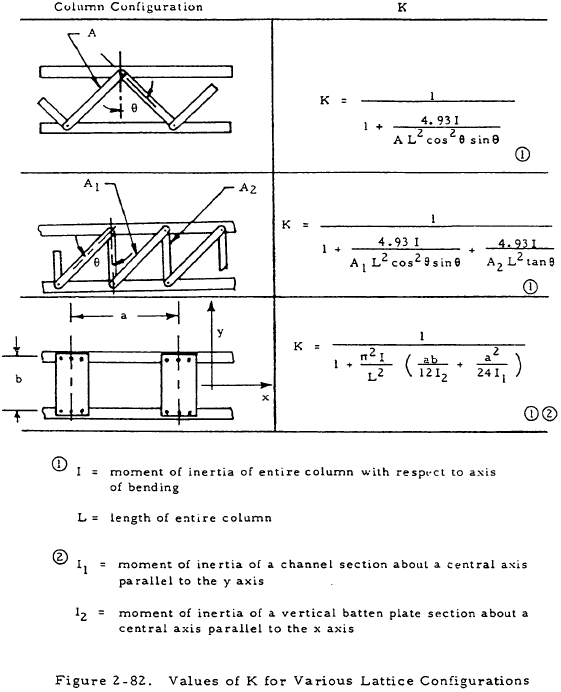﻿ Complex Columns | Engineering Library

# Analysis of Complex Columns

This page provides the sections on the analysis of complex columns from the "Stress Analysis Manual," Air Force Flight Dynamics Laboratory, October 1986.

Other related chapters from the Air Force "Stress Analysis Manual" can be seen to the right.

## Nomenclature

 A = area E = modulus of elasticity Et = tangent modulus of elasticity Fcol = maximum fiber stress for primary failure of a column f1 = stress at which the secant modulus of elasticity is equal to 0.7
 I = moment of inertia L = length n = empirical constant in Ramberg-Osgood equation P = axial load Pcr = critical load Pe = Euler critical load

## 2.4 Complex Columns

The material in previous sections treated columns that have uniform cross sections and may be considered to be one piece. This section treats of stepped and tapered columns whose cross section varies as well as of latticed columns whose action varies from that of one-piece columns.

### 2.4.1 Stepped and Tapered Columns

Columns of variable cross section can be solved by numerical procedures. However, charts are available that vastly simplify the solution of stepped and tapered columns.

These charts are shown in Figures 2-78 through 2-81. The use of these charts in finding a critical load is self-explanatory except for the fact that the tangent modulus of elasticity, Et, must be used in place of E if the section is stressed beyond the proportional limit. Also, the coefficients of constraint that were discussed in Section 2.3.1.4 no longer hold for stepped or tapered columns. The columns shown in the charts have pinned ends.

### 2.4.2 Sample Problem - Stepped Column

Given: The concentrically loaded pin ended, stepped column shown in Figure 2-77.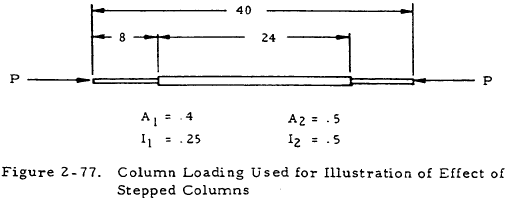Solution: Assume Pcr = 11,600 lb. Thus, Fcol.1 and Fcol.2 are equal to 11,600/0.4 psi and 11,600/0.5 psi, respectively. Equation (2-6) gives Et as

$$E_t = { E \over 1 + 3/7 ~n \sigma^{n-1} }$$

Since σ = fc /f1 where f is the compressive stress which, in this case, is Fcol,

$$E_t = { E \over 1 + 3/7 ~n \left({ F_{col} \over f_1 }\right)^{n-1} }$$

Inserting values of f1 and n from Figure 2-48 into this equation gives

$$E_t = { 10.7 \times 10^6 \over 1 + 42.9 \left({ F_{col} \over 37000 }\right)^9 }$$

Inserting the values of Fcol.1 and Fcol.2 into this equation gives

$$E_{t.1} = 1.86 \times 10^6 ~\text{psi}$$

and

$$E_{t.2} = 6.55 \times 10^6 ~\text{psi}$$

Thus

$${ E_{t.1} I_1 \over E_{t.2} I_2 } = { 1.86 \times 10^6 (0.25) \over 6.55 \times 10^6 (0.50) } = 0.142$$

From Figure 2-78, Pcr/Pe, = 0.57 where

$$P_e = { \pi^2 E_{t.2} I_2 \over L^2 }$$

Thus,

$$P_{cr} = P_e = { 0.57 \pi^2 (6.55 \times 10^6) (0.5) \over (40)^2 }$$

or Pcr = 11,600 psi. The original guess is thus correct. If it were not, other values would have to be tried until the correct value was found.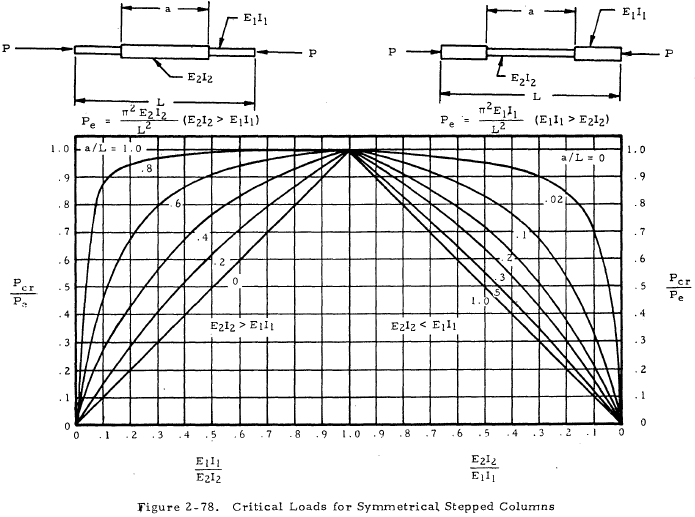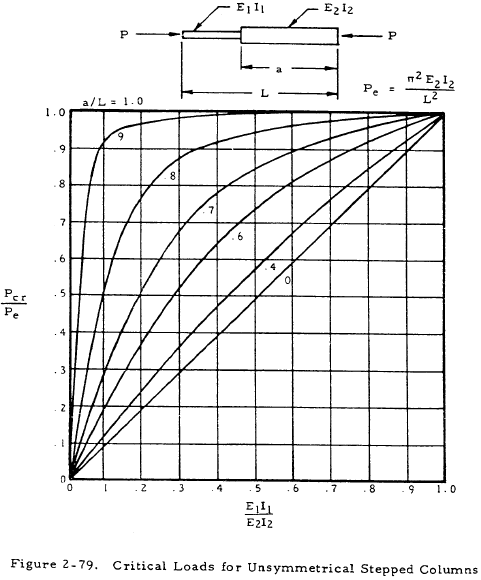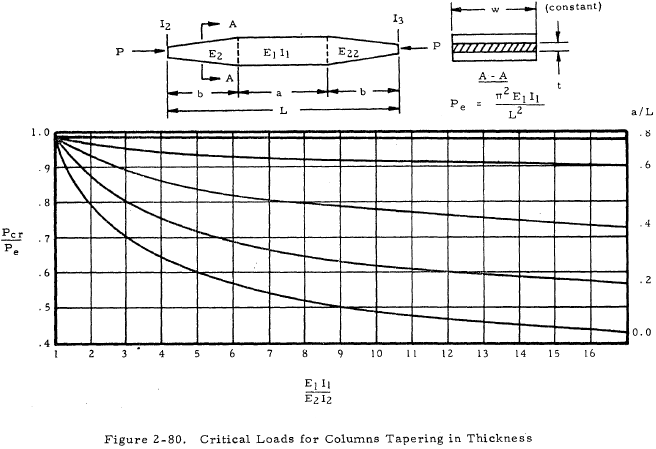### Need a Column Buckling Calculator?

Try this column buckling calculator.

• buckling analysis of long and intermediate-length columns loaded in compression